# Integer Answer Type Questions: Conic Sections | JEE Advanced - Notes | Study Maths 35 Years JEE Main & Advanced Past year Papers - JEE

 1 Crore+ students have signed up on EduRev. Have you?

1. The line 2x + y = 1 is tangent to the hyperbola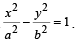If this line passes through the point of intersection of the nearest directrix and the x-axis, then the eccentricity of the hyperbola is (2010)

Ans.  (2)

Sol.  Intersection point of nearest directrix x =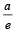and x-axis

is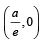As 2x +y=1 passes through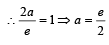Also y = –2x+1 is a tangent to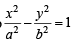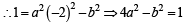⇒ 4a2– a2e2 – 1=1 ⇒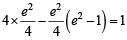⇒ 4e–e4 +e= 4 ⇒ e4 – 5e4 + 4=0

⇒ e2 = 4 ase>1 for hyperbola.  ⇒ e= 2

2. Consider the parabola y2 = 8x . Let Δ1 be the area of the triangle formed by the end points of its latus rectum and the point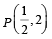on the parabola and Δ2 be the area of the triangle formed by drawing tangents at P and at the end points of the latus rectum. Then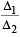is     (2011)

Ans. (2)

Sol. Δ1 =  Area of ΔPLL'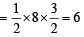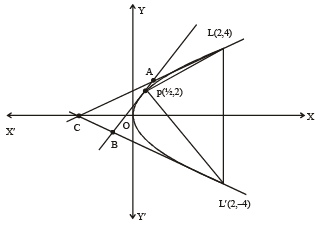Equation of AB,  y = 2x + 1 Equation of AC, y = x + 2 Equation of BC,  – y = x + 2 Solving above equations we get A (1, 3), B (–1, –1), C (–2, 0)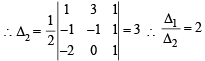3. Let S be the focus of the parabola y2  = 8x and let PQ be the common chord of the circle x2 + y2 – 2x – 4y =  0 and the given parabola. The area of the triangle PQS is     (2012)

Ans.  (4)

Sol.  We observe both parabola y2 = 8x and circle x2 + y2 – 2x – 4y = 0 pass through origin
∴ One end of common chord PQ is origin. Say P(0, 0)
Let Q be the point (2t2, 4t), then it will satisfy the equation of circle.
∴ 4t4 + 16t2 – 4t2 – 16t = 0 ⇒ t4 + 3t2 – 4t = 0  ⇒ t (t+ 3t – 4) = 0
⇒ t (t – 1)(t2 + t – 4) = 0   ⇒ t = 0 or 1
For t = 0,  we get point P, therefore t = 1 gives point  Q as (2, 4).
We also observe here that P(0, 0) and Q(2, 4) are end points of diameter of the given circle and focus of the parabola is the point S(2, 0).

∴ Area of  ΔPQS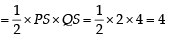sq. units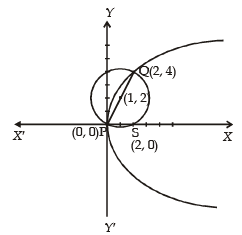4. A vertical line passing through the point (h, 0) intersects the
ellipse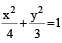at the points P and Q. Let the tangents to the ellipse at P and Q meet at the point R. If Δ(h) = area of the triangle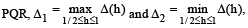then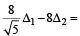(JEE Adv. 2013)
(a) g(x) is continuous but not differentiable at a
(b) g(x) is differentiable on R
(c) g(x) is continuous but not differentiable at b
(d) g(x) is continuous and differentiable at either (a) or (b) but not both

Ans. (9)

Sol. Vertical line x = h, meets the ellipse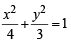at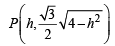and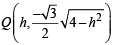By symmetry, tangents at P and Q will meet each other at x-axis.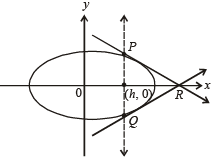Tangent at P is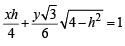which meets x-axis at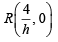Area of  ΔPQR  =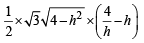i.e.,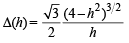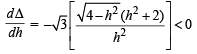∴ Δ(h) is a decreasing function.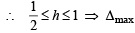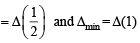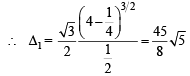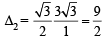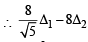= 45 – 36 = 9

5. If the normals of the parabola y2 = 4x drawn at the end points of its latus rectum are tangents to the circle (x – 3)2 + (y + 2)2 = r2, then the value of ris (JEE Adv. 2015)

Ans. (2)

Sol. End points of latus rectum of y2 = 4x are (1, +2)
Equation of normal to y= 4x at (1, 2) is y – 2 = –1(x – 1)
or x + y –3 = 0
As it is tangent to circle (x – 3)2 + (y + 2)2 = r2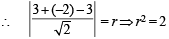6. Let the curve C be the mirror image of the parabola y2 = 4x with respect to the line x + y + 4 = 0. If A and B are the points of intersection of C with the line  y = –5, then the distance between A and B is (JEE Adv. 2015)

Ans. (4)

Sol. Let (t2, 2t) be any point on y2 = 4x. Let (h, k) be the image of (t2, 2t) in the line x + y + 4 = 0. Then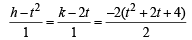⇒ h = –(2t + 4) and k = –(t2 + 4)
For its intersection with, y = –5, we have –(t2 + 4) = –5 ⇒ t = +1
∴ A(–6, –5) and B(–2, –5) ∴ AB = 4.

7. Suppose that the foci of the ellipse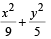= 1 are (f1, 0)and (f2, 0) where f1 > 0 and f2 < 0. Let P1 and P2 be two parabolas with a common vertex at (0, 0) and with foci at (f1, 0) and (2f2, 0), respectively. Let T1 be a tangent to P1 which passes through (2f2, 0) and Tbe a tangent to P2 which passes through (f1, 0). If m1 is the slope of T1 and m2 is the slope of T2, then the value of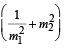is (JEE Adv. 2015)

Ans. (4)

Sol.  Ellipse :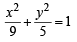⇒ a = 3, b =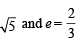∴ f1 = 2 and f= –2 P1 : y2 = 8x and P2 : y2 = –16x

T1 : y = m1x  +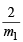It passes through (–4, 0),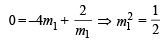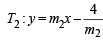It passes through (2, 0)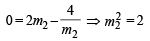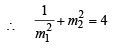The document Integer Answer Type Questions: Conic Sections | JEE Advanced - Notes | Study Maths 35 Years JEE Main & Advanced Past year Papers - JEE is a part of the JEE Course Maths 35 Years JEE Main & Advanced Past year Papers.
All you need of JEE at this link: JEE

## Maths 35 Years JEE Main & Advanced Past year Papers

132 docs|70 tests
 Use Code STAYHOME200 and get INR 200 additional OFF

## Maths 35 Years JEE Main & Advanced Past year Papers

132 docs|70 tests

Track your progress, build streaks, highlight & save important lessons and more!

,

,

,

,

,

,

,

,

,

,

,

,

,

,

,

,

,

,

,

,

,

;